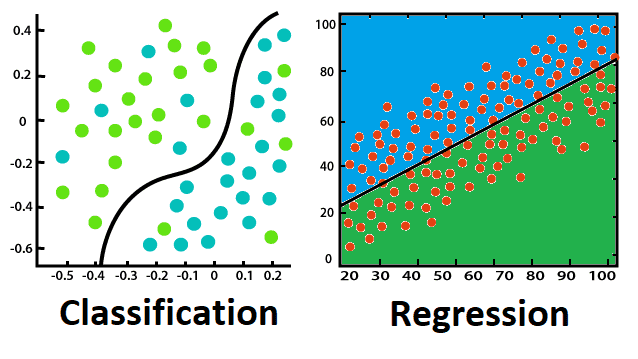# Regression vs Classification in Machine Learning

In this page Regression vs Classification in Machine Learning, Types of ML Classification Algorithms, Classification Algorithms can be further divided into the following types, Types of Regression Algorithm, Difference between Regression Algorithm and Classification Algorithm.

Supervised Learning algorithms include regression and classification techniques. Both techniques are used in Machine Learning for prediction and work with labeled datasets. The distinction between the two is how they're applied to various machine learning situations.
The main difference between Regression and Classification algorithms is that Regression algorithms are used to predict continuous values like price, salary, age, and so on, whereas Classification algorithms are used to predict discrete values like Male or Female, True or False, Spam or Not Spam, and so on.
Consider the below diagram:## Classification:

Classification is the process of identifying a function that aids in the classification of a dataset based on several factors. A computer program is trained on the training dataset and then categorizes the data into distinct classes based on that training.
The classification algorithm's goal is to identify the mapping function that will convert the discrete input(x) to the discrete output(y).
Example: Email Spam Detection is the finest example for understanding the Classification challenge. The model is trained on millions of emails on various parameters, and it determines if an email is spam or not when it receives a new one. The email is moved to the Spam folder if it is spam.

## Classification Algorithms can be further divided into the following types:

• Logistic Regression
• K-Nearest Neighbours
• Support Vector Machines
• Kernel SVM
• Naïve Bayes
• Decision Tree Classification
• Random Forest Classification

## Regression:

Regression is a process of finding the relationships between dependent and independent variables. It aids in the prediction of continuous variables such as market trends, house values, and so forth.
The Regression algorithm's goal is to identify the mapping function that will translate the continuous input variable (x) to the discrete output variable (y) (y).
For example, let's say we want to forecast the weather, so we'll apply the Regression algorithm. When it comes to weather prediction, the model is trained on historical data, and after it is finished, it can accurately predict the weather for future days.

## Types of Regression Algorithm:

• Simple Linear Regression
• Multiple Linear Regression
• Polynomial Regression
• Support Vector Regression
• Decision Tree Regression
• Random Forest Regression

## Difference between Regression Algorithm and Classification Algorithm

Regression Algorithm Classification Algorithm
The output variable in regression must be continuous or have a genuine value. The output variable in classification must be a discrete value.
The regression algorithm's job is to map the continuous output variable (y) to the input value (x) (y). The classification algorithm's job is to map the discrete output variable to the input value(x) (y).
Continuous data is used with regression algorithms. With discrete data, classification algorithms are applied.
In regression, we strive to identify the best fit line that can more accurately predict the output. We aim to find the decision boundary in classification to divide the dataset into various classes.
Weather prediction, house price prediction, and other regression problems can be solved using regression algorithms. Classification algorithms can be used to handle problems like identifying spam emails, speech recognition, and cancer cell identification, among others.
Linear and non-linear regression algorithms are two types of regression algorithms. Binary Classifier and Multi-class Classifier are two types of classification algorithms.